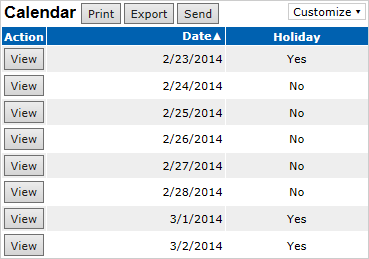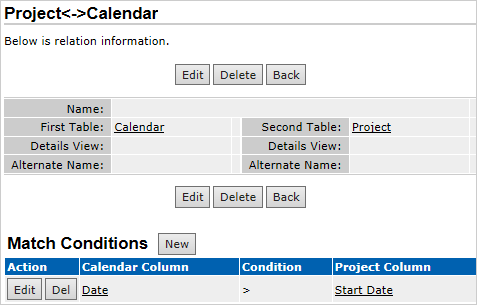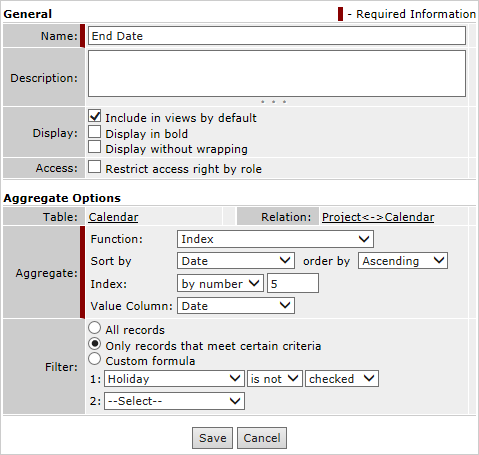Calculating future dates (such as exact due dates or project delivery dates) is a pretty common task.

Let’s find an easy way to calculate future dates in TeamDesk.

The simplest way is to add a number of days to a date value. For example, the End Date is 5 days after the Start Date. You can use the following formula for the End Date calculation:

[Start Date]+5d

The problem can occur if you need to account working days only, while excluding weekends and holidays from calculation.

In this case you need to create the Calendar table. It has to comprise the Date column of the date type and the Holiday column of the checkbox type. The Date column must be set as a key column of the Calendar table.

Now you can import the list of current year dates into the Calendar table. It’s handier to import an excel file with dates. Then mark weekends and holidays in the Holiday checkbox column.Create a Many-To-Many relation with a match condition [Date] > [Start Date]Create the End Date summary column with the Index function and filters.As the result the End Date will be calculated automatically according to the data stored in the Calendar table.

Author
Date
Share